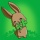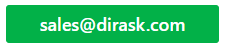Home
IT Knowledge
IT Career
Inspiration
Languages
EN

# C# / .NET - fastest way to negate number

6 points
Created by:JustMike
26120

In C# / .NET it is possible to negate numbers in following ways:

1. `x = -x`
2. `x *= -1`
3. `x = ~x + 1`

## 1. Complexity analysis

1. `x = -x` operations:
2. make value negation
3. write value to memory
2. `x *= -1` (`x = x * (-1)`) operations:
2. make multiplication by `-1` operation
3. write value to memory
3. `x = ~x + 1` operations:
2. make bitwise not operation
3. make add `+1` operation
4. write value to memory

Notes:

• multiplication operations are slower than bitwise and subtract operations,
• in this case interpreter / compliler can make some optimisation,
• amount of operations affects on perfomrance,
• getting and setting variables from RAM memory can be slow.

The best way is always to make a benchmark test.

## 2. Benchmark test example

``````using System;
using System.Diagnostics;

public class Program
{
public class Result
{
public String Name;
public long DT;

public Result(String name, long dt)
{
this.Name = name;
this.DT = dt;
}
}

//
public static long GetNanoseconds()
{
double timestamp = Stopwatch.GetTimestamp();
double nanoseconds = 1000000000.0 * timestamp / Stopwatch.Frequency;

return (long)nanoseconds;
}

public void Main(String[] args)
{
// configutarion

int testsCount = 1;
int testSize = 100000000;

// test

Random random = new Random();

Result result1 = new Result("x = -x;", 0);
Result result2 = new Result("x *= -1;", 0);
Result result3 = new Result("x = ~x + 1;", 0);

long t1, t2;
long x = random.Next();

for (int i = 0; i < testsCount; ++i)
{
// case 1
t1 = GetNanoseconds();

for (int j = 0; j < testSize; ++j)
x = -x;

t2 = GetNanoseconds();
result1.DT += t2 - t1;

// case 2
t1 = GetNanoseconds();

for (int j = 0; j < testSize; ++j)
x *= -1;

t2 = GetNanoseconds();
result2.DT += t2 - t1;

// case 3
t1 = GetNanoseconds();

for (int j = 0; j < testSize; ++j)
x = ~x + 1;

t2 = GetNanoseconds();
result3.DT += t2 - t1;
}

// summary

Result[] results = { result1, result2, result3 };
Result max = results;

for (int i = 1; i < results.Length; ++i)
{
Result result = results[i];

if (result.DT > max.DT)
max = result;
}

Console.WriteLine("x=" + x);

//Console.WriteLine(result1.Name + " // " + (result1.DT / 1E+6)+ "ms");
//Console.WriteLine(result2.Name + " // " + (result2.DT / 1E+6) + "ms");
//Console.WriteLine(result3.Name + " // " + (result3.DT / 1E+6) + "ms");

for (int i = 0; i < results.Length; ++i)
{
Result result = results[i];

if(result == max)
{
Console.WriteLine(result.Name
+ " // " + (result.DT / 1E+6) + "ms");
}
else
{
double imprDifference = max.DT - result.DT; // improvement
double imprPercentage = 100 * imprDifference / max.DT;

String imprText = String.Format("{0:0.00}", imprPercentage);

Console.WriteLine(result.Name
+ " // " + (result.DT / 1E+6) + "ms"
+ " -> " + imprText  + "% faster than " + max.Name);
}
}
}
}``````

Output (.NET 4.7.2):

``````x=1598783071
x = -x; // 294.8186ms -> 57.15% faster than x *= -1;
x *= -1; // 688.0959ms
x = ~x + 1; // 305.058399ms -> 55.67% faster than x *= -1;``````